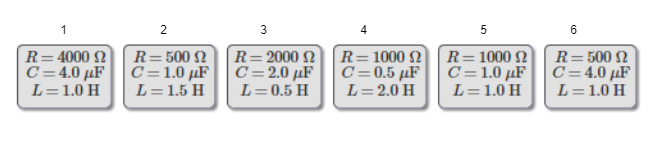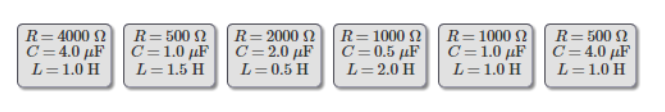# Problem: Rank these circuits on the basis of their resonance frequencies.

###### FREE Expert Solution

Resonance frequency:

$\overline{){\mathbf{f}}{\mathbf{=}}\frac{\mathbf{1}}{\mathbf{2}\mathbf{\pi }\sqrt{\mathbf{L}\mathbf{C}}}}$

Let's label the circuits as follows:84% (294 ratings)###### Problem Details

Rank these circuits on the basis of their resonance frequencies.